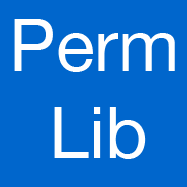# User's Guide for PermLib

## INTRODUCTION:

PermLib is a C++ library for permutation computations. Currently it supports set stabilizer and in-orbit computations, based on bases and strong generating sets (BSGS). Additionally, it computes automorphisms of symmetric matrices and find the lexicographically smallest set in an orbit of sets. It also features a very basic recognition of permutation group types. You may download the complete PermLib package (version 0.2.8) and then follow the instructions below. Additional background information about the implementation can be found in the Diploma thesis of the author Thomas Rehn.

Code repository and issue tracker on github.

## USAGE:

PermLib currently has five main directories:
• `data` contains various permutation groups for testing or benchmarking.
• `doc` contains automatically generated documentation.
• `example` contains examples and the applications which were used to benchmark PermLib.
• `include` contains the PermLib core. PermLib is completely implemented in C++ header files.
• `test` contains various unit tests.

The only dependency to build PermLib is Boost in version 1.34.1 or higher. However, the build system for the contributed applications makes use of CMake. If CMake and Boostare correctly installed the PermLib applications can be built with:

```~/permlib\$ mkdir build && cd build
~/permlib/build\$ cmake -DCMAKE_BUILD_TYPE=RelWithDebInfo ..
~/permlib/build\$ make
```
Then, for instance, `./example/example` should run the example application below and compute a set stabilizer.

CMake is not required to use PermLib. Only the `include` directory has to be included in your project.

Version 0.2.1 introduces a new compact API to access the core functionality of PermLib. It is available from the `<permlib/permlib_api.h>` include. The API example below, which is also `api-example.cpp` from the `example` folder, illustrates its features and usage. For instance, this example file could be compiled as a stand-alone application with a GNU C++ compiler as

``` g++ -I../include -o api-example api-example.cpp
```

## REFERENCES:

PermLib is currently used by the following projects:

• Polymake: action of (symmetry) groups on polyhedra
• SymPol: polyhedral description conversion up to symmetries

If you are using PermLib in your code, please write an email to thomas.rehn (aatt) uni-rostock.de. If you want to give a scientific reference to PermLib, consider citing [RS10].

## EXAMPLE:

```#include <permlib/permlib_api.h>

#include <iostream>

int main(int argc, char *argv[]) {
using namespace permlib;

// our group will have degree 10, i.e. act on 1..10
const ulong n = 10;

// group generators
PERMlist groupGenerators;

boost::shared_ptr<Permutation> gen1(new Permutation(n, std::string("1 3 5 7 9 10 2 4 6 8")));

groupGenerators.push_back(gen1);
boost::shared_ptr<Permutation> gen2(new Permutation(n, std::string("1 5")));

groupGenerators.push_back(gen2);

//
// EXAMPLE 0 (BSGS): construct a base with strong generating set
//
boost::shared_ptr<PermutationGroup> group = construct(n, groupGenerators.begin(), groupGenerators.end());

std::cout << "Group " << *group << std::endl;

// size of our set(s) in this example
const ulong DeltaSize = 4;
// represents the set {1,5,8,9}, translated by -1 as the elements of the domain are 0-based
const ulong Delta[DeltaSize] = {0, 4, 7, 8};

//
// EXAMPLE 1 (SET STAB): compute a set stabilizer
//
boost::shared_ptr<PermutationGroup> stabilizer = setStabilizer(*group, Delta, Delta+DeltaSize);

std::cout << "Stabilizer of {1,5,8,9}: " << *stabilizer << std::endl;

//
// EXAMPLE 2 (SET IMAGE): find elements mapping one set onto another
//
const ulong Gamma[DeltaSize] = {2, 6, 0, 9};

boost::shared_ptr<Permutation> repr = setImage(*group, Delta, Delta+DeltaSize, Gamma, Gamma+DeltaSize);

if (repr)
std::cout << "Group element mapping {1,5,8,9} to {1,3,7,10}: " << *repr << std::endl;

else
std::cout << "No group element found mapping {1,5,8,9} to {1,3,7,10}." << std::endl;

const ulong Gamma2[DeltaSize] = {2, 6, 10, 9};

boost::shared_ptr<Permutation> repr2 = setImage(*group, Delta, Delta+DeltaSize, Gamma2, Gamma2+DeltaSize);

if (repr2)
std::cout << "Group element mapping {1,5,8,9} to {3,7,10,11}: " << *repr2 << std::endl;

else
std::cout << "No group element found mapping {1,5,8,9} to {3,7,10,11}." << std::endl;

//
// EXAMPLE 3 (ORBTIS): compute orbits of a group
//                     in this case: the stabilizer from above
//
std::list<boost::shared_ptr<OrbitAsSet> > orbitList = orbits(*stabilizer);

ulong orbCount = 1;
BOOST_FOREACH(const boost::shared_ptr<OrbitAsSet>& orbit, orbitList) {

std::cout << "Orbit #" << orbCount << " representative: " << (orbit->element()+1) << std::endl;
++orbCount;
}

//
// EXAMPLE 4 (SMALLEST SET IMAGE): compute lexicographically smallest set of an orbit of sets
//
// encode Gamma in a 'dset', which is a boost::dynamic_bitset
dset dGamma(n);
for (uint i = 0; i < DeltaSize; ++i)

dGamma.set(Gamma[i]);
dset dGammaLeast = smallestSetImage(*group, dGamma);

std::cout << "Lexicographically smallest set in the orbit of {1,3,7,10}:  {";
for (uint i = 0; i < n; ++i)

if (dGammaLeast[i])
std::cout << (i+1) << ",";

std::cout << "}" << std::endl;

return 0;
}

```

## REFERENCES:

[HEO05]
Derek F. Holt, Bettina Eick, and Eamonn A. O'Brien. Handbook of Computational Group Theory. Discrete Mathematics and Applications. Chapman & Hall/CRC, 2005.
[Leo91]
Jeffrey S. Leon. Permutation group algorithms based on partitions, I: Theory and algorithms. Journal of Symbolic Computation, 12:533–583, 1991. [DOI]
[Reh10]
Thomas Rehn. Fundamental Permutation Group Algorithms for Symmetry Computation. Diploma thesis (computer science), Otto von Guericke University Magdeburg, February 2010. [PDF]
[RS10]
Thomas Rehn, Achill Schürmann. C++ Tools for Exploiting Polyhedral Symmetries. Lecture Notes in Computer Science, 2010, Volume 6327/2010. [PDF]

## CONTACT:

If you have any kind of question about PermLib, it's usage or you have additions, remarks, comments, etc. please feel free to contact its author Thomas Rehn and/or Achill Schürmann
Last update: Sep 27 2012